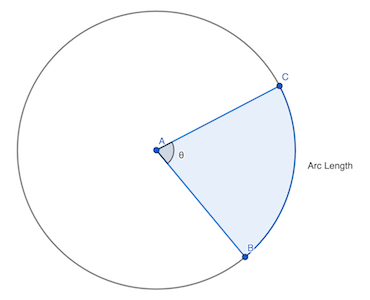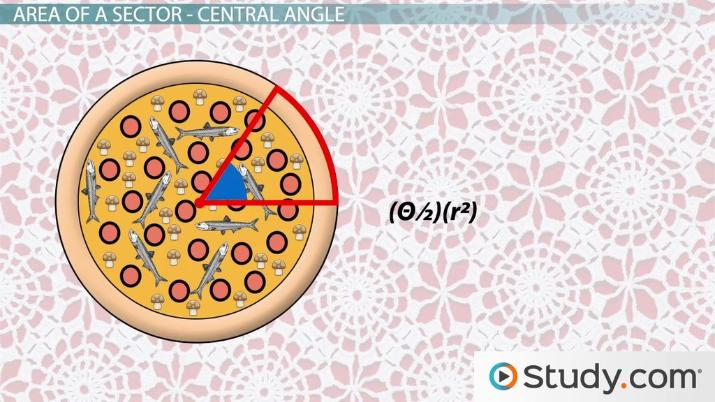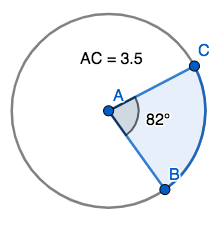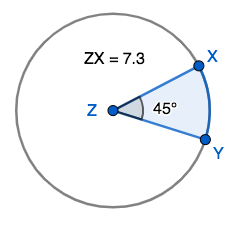# Arc Length and Sector Area

Roberto Robert Santiago, Jeff Calareso
• Author
Roberto Robert Santiago

Roberto has worked for 10 years as an educator: six of them teaching 5th grade Math to Precalculus in Puerto Rico and four of them in Arizona as a Middle School teacher. I also have a BA Degree in Secondary Education from the University of Puerto Rico, Rio Piedras Campus.

• Instructor
Jeff Calareso

Jeff teaches high school English, math and other subjects. He has a master's degree in writing and literature.

Learn how to find the arc length of a sector with the formula and examples. Understand the formula and the method to find the area of a sector with examples. Updated: 12/29/2021

Show

## Arc Length and Sector Area

When working with circles, you may come across a situation where you do not need to know the entire circumference of it, only the arc length between two points around it. Generally speaking, an arc of a circle is a smooth curved bounded by two distinct points. Alternatively, any arc is a portion of the circumference of a circle, bounded by two points, rather than the entire circle. Arc length is the distance of the arc bounded by two points. A sector of a circle is the area that is between the two radii of the circle that uses the endpoints of the arc.In the above image, the endpoints of arc BC are connecting from center point A, forming radii AC and AB. The sector is the area between those two radii.An error occurred trying to load this video.

Try refreshing the page, or contact customer support.

Coming up next: Constructing Inscribed & Circumscribed Triangles

### You're on a roll. Keep up the good work!

Replay
Your next lesson will play in 10 seconds
• 0:07 Circles
• 0:39 What is a Sector?
• 1:45 Area of Sector - Central Angle
• 3:27 Arc Length
• 4:29 Area of Sector - Arc Length
• 5:22 Lesson Summary
Save Save

Want to watch this again later?

Timeline
Autoplay
Autoplay
Speed Speed

## Arc Length of a Sector Formula

To find the length of the arc, we need two things: the length of the radius and the measure of the central angle. We will label arc BC as {eq}s {/eq}. Additionally, we need the length of one of the radii ({eq}r {/eq}) and the measure of the central angle (the angle whose vertex is at the center of the circle), which we will label as {eq}\theta {/eq}. The formula looks like this: $$s=r\theta$$Keep in mind that the measure of the central angle must be expressed in radians rather than in degrees. To convert from degrees to radians use the following formula:

$$\theta= d^o( \frac{\pi}{180^o})$$ where {eq}d^o {/eq} is the measure of the central angle, in degrees. If we were to substitute this piece by {eq}\theta {/eq} we would have this expanded form of the arc length formula:

$$s=rd( \frac{\pi}{180})$$.

## How to Find the Arc Length of a Sector.

1. Convert angle measure to radian measure, if necessary.
2. Multiply the radian measure by the length of the radius and round the product to the desired number place.

### Example 1: Find the length of arc BC, as shown on the diagram below. Assume the length measures are in centimeters. Round to the nearest tenth of a centimeter.First, we identify our angle measure and convert it into radians. In this example, the angle measure is 82 degrees, so we must convert that into radians as shown below:

$$\theta = 82(\frac{\pi}{180})$$ We then simplify our fraction {eq}\frac{82}{180} {/eq}: {eq}\pi {/eq}, leading to this measure in radians:

$$\theta=\frac{41}{90}\pi$$ Now we plug in the angle measure and our radius measure in the arc length formula and calculate the length:

$$s=r\theta$$ $$s=3.5(\frac{41}{90}\pi)$$$$s\approx3.5(0.4556)(3.14)$$$$s\approx5.0$$

Therefore, the length of arc BC is about 5.0 centimeters.

### Example 2: Calculate arc length XY using the information on the diagram below. Round to the nearest tenth is possible.To unlock this lesson you must be a Study.com Member.

#### What is the formula for arc length of a sector?

The formula to find the arc length of a sector is as follows: {eq}s=r\theta {/eq}, where you would need the length of the radius between the endpoint of the arc and the center of the circle, and the angle's measure in radians.

#### What is the formula for area of the sector?

The formula to calculate the area of the sector is $$A=\frac{\theta}{360^o}\times{\pi}r^2$$, where you would need the measure of the central angle in degrees and the radius length.

#### How do you find arc length with angle and radius?

To find arc length given an angle measure and a radius, you would need to first convert the angle measure into radians, then multiply that number by the length of the radius.

### Register to view this lesson

Are you a student or a teacher?